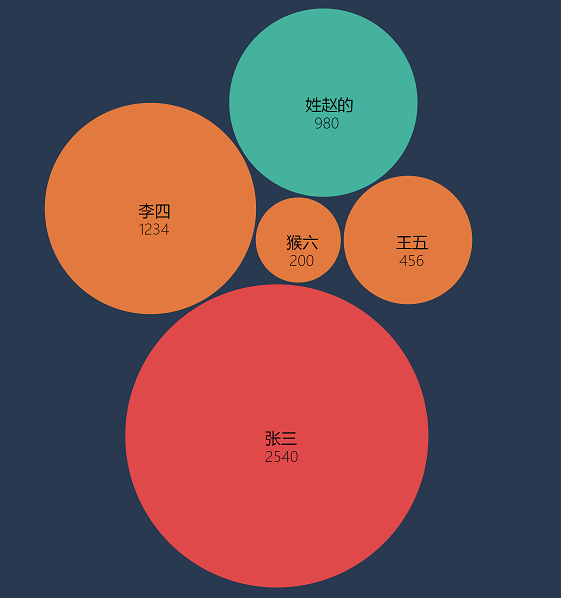# 波斯码BOSSMA Information Technology

## 如何制作漂亮的”气泡云图”ECharts、Highcharts都没有提供这种图表展现形式（或者是我没找到？）。

1、首先定义一个html页面

```<html>
<script src="https://d3js.org/d3.v3.min.js" type="text/javascript"></script>
<body>
<h1>D3 Bubble Chart</h1>
<section id="graph"></section>
</body>
</html>```

2、然后就是重点部分了

```(function() {

// 要展现的数据，name为主题名称，className为气泡的样式，size将用于气泡大小
var dataSet = [];
dataSet.push({name: '张三', className: 'item0', size: 2540});
dataSet.push({name: '李四', className: 'item1', size: 1234});
dataSet.push({name: '王五', className: 'item2', size: 456});
dataSet.push({name: '猴六', className: 'item3', size: 200});
dataSet.push({name: '姓赵的', className: 'other', size: 980});

// 将数据包装下层次
var json = {children: dataSet}

// 图形区域大小
var diameter = 600;

// 在id=graph的section创建SVG区域
var svg = d3.select('#graph').append('svg')
.attr('width', diameter)
.attr('height', diameter);

// 用递归的圆-包生成一个层次布局，建议取消sort注释看看
var bubble = d3.layout.pack()
.size([diameter, diameter])
.value(function(d) {return d.size;})
// .sort(function(a, b) {
// 	return -(a.value - b.value)
// })

// 计算包布局并返回节点数组
var nodes = bubble.nodes(json)
.filter(function(d) {
return !d.children;
});

// 绑定气泡元素和数据
var vis = svg.selectAll('g').data(nodes);

// 定义气泡
var node = vis.enter().append("g")
.attr("class", "node")
.attr("transform", function(d) { return "translate(" + d.x + "," + d.y + ")"; });

// 画气泡（圆圈）
node.append("circle")
.attr("r", function(d) { return d.r; }) //圆的半径
.attr('class', function(d) { return d.className; }); //圆的颜色

// 设置气泡（圆圈）上的文字
node.append("text")
.selectAll("tspan") // text下的文字用tspan包裹，这里将创建两个
.data(function(d) { return [d.name,d.size]; }) // text下的两行文字数据：主题名称和数据大小
.enter().append("tspan") // 这里会创建两个tspan
.attr("x", function(d, i) { // 文字的水平位置，大代表当前tspan文字内容
if(isNaN(d)){
return 0-(d.length/2)*12;
}else{
return 0-(d.toString().length/2)*6; // 数字字符的宽度短一些
}
})
.attr("y", function(d, i) { // 文字的垂直位置，i代表第几个tspan
return 8 + i * 18;
})
.text(function(d) { return d; }); // 文字的内容

// 鼠标划过显示
node.append("title")
.text(function(d) { return d.name+"\n"+d.size; });
})();```

```// 画气泡（圆圈）
node.append("circle")
.attr("r", function(d) { return d.r; }) //圆的半径
.style("fill", function(d) { return '#aabbcc'; }); //圆的颜色
```

### 发表评论